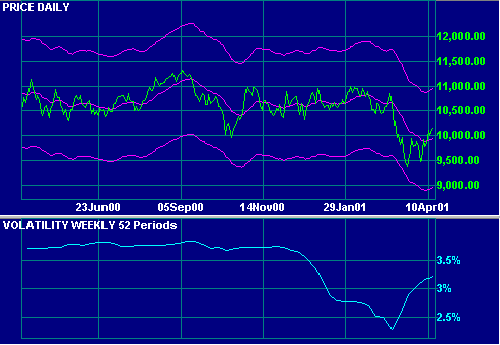# Volatility Indicator

This is a statistical measure of volatility called the coefficient of variation. It measures the standard deviation of closing price from its simple moving average.

Volatility is normally used to measure the risk profile of managed funds.

## Example

Dow Jones Industrial Average with      52 week Volatility.## Setup

The default period is 125 days. To alter the default settings - Edit Indicator Settings.

See Indicator Panel for directions on how to set up an indicator.

## Volatility Indicator Formula

Volatility = standard deviation of closing price [for n periods] / average closing price [for n periods]

n periods is normally taken for 1 to 5 years.

### Standard Deviation

For the more technically-minded, Standard Deviation is the basic statistical measure of the dispersion of a population of data observations around a mean. Referred to as STD (or by the Greek symbol sigma) it is the square root of the Variance.

To calculate the Variance:

1. Measure the deviation of each data point from the Mean (the average of the entire population).
2. Square the deviations.
3. Sum the squared deviations.
4. Divide the sum by the total number of observations.

STD is calculated as the square root of the Variance.